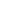# Free CBSE Class 9 Mathematics Unit 5-Mensuration Heron's formula Worksheets

Download free printable Heron's formula Worksheets to practice. With thousands of questions available, you can generate as many Heron's formula Worksheets as you want.

## Sample CBSE Class 9 Mathematics Unit 5-Mensuration Heron's formula Worksheet Questions

1.

A cuboidal oil tin is 30 cm X 40 cm X 50 cm. Find the cost of the tin required for making 20 such tins if the cost of tin sheet is .

2.

The dimensions of a cubiod are in the ratio of 1 : 2 : 3 and its total surface area is . Find the dimensions.

3.

Three metal cubes whose edge measure 3 cm, 4 cm and 5 cm respectively are melted to form a single cube, find its edge.

4.

The diameter of a garden roller is 1.4 m and it is 2 m long. How much area will it cover in 5 revolutions?

5.

A solid cylinder has a total surface area of . Its curved surface area is of the total surface area. Find the volume of the cylinder.

6.

Find the volume of a sphere whose surface area is .

7.

A triangle and a parallelogram have the same base and some area. If the sides of the triangle are 26 cm, 28 cm and 30 cm and the parallelogram stands on the base 28 cm, find the height of the parallelogram.

8.

An umbrella is made by stitching 10 triangular pieces of cloth of 5 different colour each piece measuring 20 cm, 50 cm and 50 cm. How much cloth of each colour is required for one umbrella?

9.

A field is in the shape of a trapezium whose parallel sides are 25 m and 10 m. If its non-parallel sides are 14 m and 13 m, find its area.

10.

The perimeter of a triangular ground is 420 m and its sides are in the ratio 6 : 7 : 8. Find the area of the triangular ground.

## Find more Heron's formula Worksheets

Worksheets by UrbanPro

Our worksheets are designed to help students explore various topics, practice skills and enrich their subject knowledge, to improve their academic performance. Designed by Experts who have extensive experience and expertise in teaching a subject, these worksheets will improve your child's problem-solving skills and subject knowledge in a fun and interactive manner.
Check out our free customized worksheets across school boards, grades, subjects and levels of subject knowledge. You can download, print and share these worksheets with anyone, anywhere, anytime!

Get a custom worksheet to practice!

Select your topic & see the magic.

subjectSelect Chapter(s)

Chapters & Subtopics